# Texas Go Math Grade 2 Lesson 10.6 Answer Key 3-Digit Subtraction: Regroup Hundreds

Refer to our Texas Go Math Grade 2 Answer Key Pdf to score good marks in the exams. Test yourself by practicing the problems from Texas Go Math Grade 2 Lesson 10.6 Answer Key 3-Digit Subtraction: Regroup Hundreds.

## Texas Go Math Grade 2 Lesson 10.6 Answer Key 3-Digit Subtraction: Regroup Hundreds

Explore

Draw quick pictures to show the problem.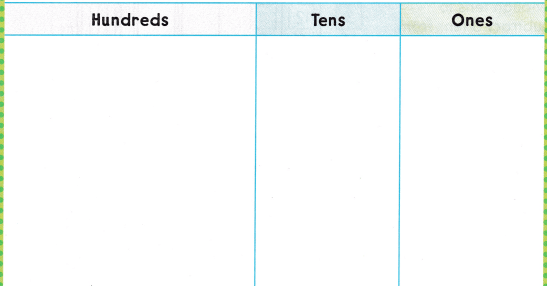FOR THE TEACHER • Read the following problem and have children model it with quick pictures. The Reading Club collected 349 books 173 books were fiction books. The other books were nonfiction books. How many nonfiction books were there?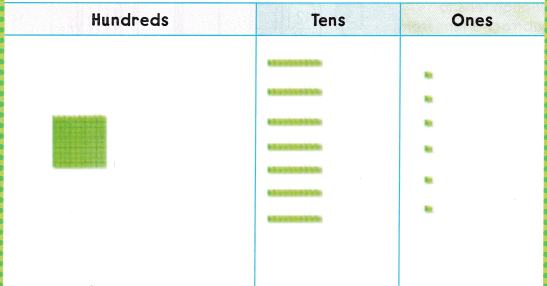Explanation:
The difference of 349 and 173 is 176.

Math Talk
Mathematical Processes

Describe what to do when there are not enough tens to subtract from.

Model and DrawShare and Show

Solve. Write the difference.

Question 1.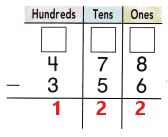Explanation:
The difference of 478 and 356 is 122.

Question 2.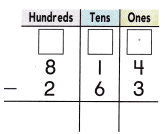Explanation:
The difference of 814 and 263 is 551.

Problem Solving

Solve. Write the difference.

Question 3.Explanation:
The difference of 435 and 192 is 243.

Question 4.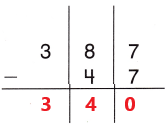Explanation:
The difference of 387 and 47 is 340.

Question 5.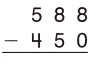Explanation:
The difference of 588 and 450 is 138.

Question 6.Explanation:
The difference of 345 and 263 is 82.

Solve. Write or draw to explain.

Question 7.
H.O.T. Multi-Step Sam built two towers. He used 139 blocks for the first tower. He used 276 blocks in ail. For which tower did he use more blocks?Explain how you solved the problem.
276-139=137

Explanation:
Sam built two towers.
He used 139 blocks for the first tower.
He used 276 blocks in ail.
For second tower he uses more blocks that is 276.

Question 8.
H.O.T. There were 375 puzzle pieces in the box. Liz took 190 pieces out of the box. 95 of those pieces are placed. How many puzzles pieces are still in the box?__________ puzzle pieces
375-190+95=280
280 puzzle pieces

Explanation:
There were 375 puzzle pieces in the box.
Liz took 190 pieces out of the box.
95 of those pieces are placed.
280 puzzles pieces are still in the box.

Question 9.
Janice needs 225 blocks to build a model of a house. She has 182 blocks. How many more blocks does Janice need?
(A) 52
(B) 43
(C) 47
(B)43

Explanation:
Janice needs 225 blocks to build a model of a house.
She has 182 blocks.
225-182=43
More 43 blocks Janice need to model a house.

Question 10.
Reasoning A class has 175 building logs. The teacher brought in some more logs, and now the class has 318 logs. How many building logs did the teacher bring?
(A) 143
(B) 233
(C) 133
(A)143

Explanation:
Reasoning A class has 175 building logs.
The teacher brought in some more logs, and now the class has 318 logs.
318-175=143
The teacher brings 143 building logs.

Question 11.
Analyze A model of a ship is made of 495 pieces. Some are metal and some are wooden. There are 375 wooden pieces. How many pieces are metal?
(A) 75
(B) 120
(C) 70
(B)120

Explanation:
A model of a ship is made of 495 pieces.
Some are metal and some are wooden.
There are 375 wooden pieces.
495-375=120
120 pieces are metal.

Question 12.
TEXAS Test Prep Mr. Simms has 315 paper hats. He gives 140 paper hats to students in the parade. How many paper hats does he have now?(A) 235
(B) 255
(C) 175
(C)175

Explanation:
Mr. Simms has 315 paper hats.
He gives 140 paper hats to students in the parade.
315-140=175
175 paper hats he have now.

TAKE HOME ACTIVITY • Have your child explain how to find the difference for 745 – 341.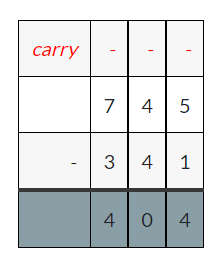Explanation:
The difference of 745 and 341 is 404.

### Texas Go Math Grade 2 Lesson 10.6 Homework and Practice Answer Key

Solve. Write the difference.

Question 1.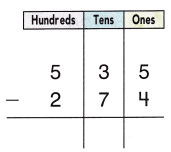Explanation:
The difference of 535 and 274 is 261.

Question 2.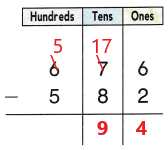Explanation:
The difference of 676 and 582 is 94.

Question 3.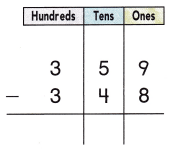Explanation:
The difference of 359 and 348 is 11.

Question 4.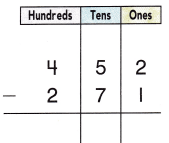Explanation:
The difference of 452 and 271 is 81.

Problem Solving

Solve. Write or draw to explain.

Question 5.
Multi-Step Greg built a stone wall. He used 374 stones in all. He used 232 stones along the long side. He used the rest for the short side of the wall. On which side of the wail did he use more stones? How can you tell?
374-232=142

Explanation:
Multi-Step Greg built a stone wall.
He used 374 stones in all.
He used 232 stones along the long side.
He used the rest for the short side of the wall.
374-232=142
So, he uses more stones for long side of he wall.

Lesson Check

Question 6.
Bob’s Party Shop has 250 balloons. He gave 135 balloons to children for a school play. How many balloons does he have now?
(A) 385
(B) 115
(C) 125
(B)115

Explanation:
Bob’s Party Shop has 250 balloons.
He gave 135 balloons to children for a school play.
250-135=115
115 balloons he have now.

Question 7.
A model of a house is made of 525 pieces. Some are round and some are square. There are 450 square pieces. How many pieces are round?
(A) 75
(B) 25
(C) 175
(A)75

Explanation:
A model of a house is made of 525 pieces.
Some are round and some are square. There are 450 square pieces.
525-450=75
75 pieces are round.

Question 8.
James is building a model house with bricks and logs from a kit. The kit has 458 pieces. James has used 160 pieces so far. How many more pieces are left in the kit?
(A) 292
(B) 618
(C) 298
(C)298

Explanation:
A model of a house is made of 525 pieces.
Some are round and some are square.
There are 450 square pieces.
525-450=298
298 pieces are round.

Question 9.
There are 185 books in Ms. Casey’s classroom. Ms. Casey received some more books. Now there are 265 books in the classroom. How many books did Ms. Casey receive?
(A) 120
(B) 70
(C) 80